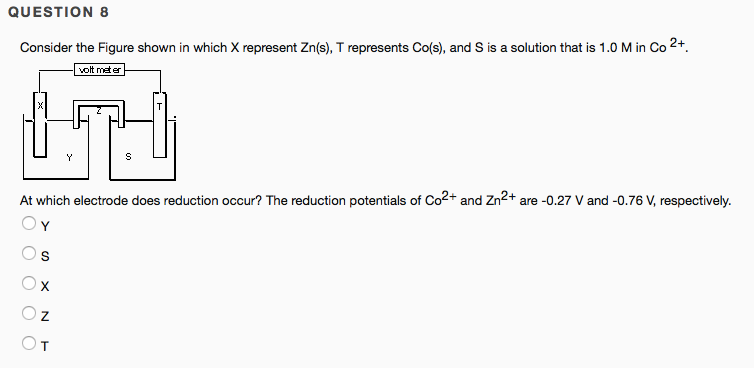# Consider the Figure shown in which X represent Zn(s), T represents Co(s), and S is a solution that is 1.00 M in Co^2+. At which electrode does reduction occur? The reduction potentials of Co^2+ and Zn^2+ are -0.27 V and -0.76 V, respectively. a) Y b) S c) X d) Z e) T